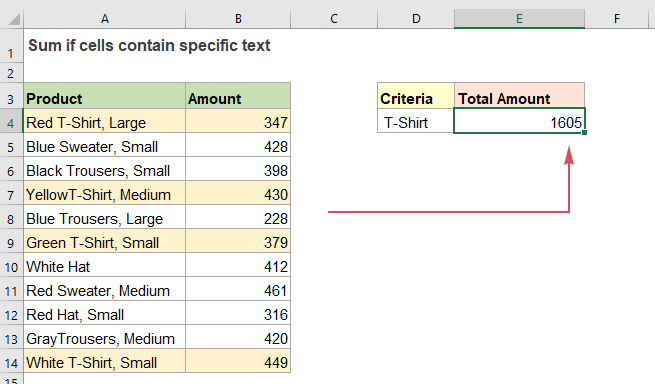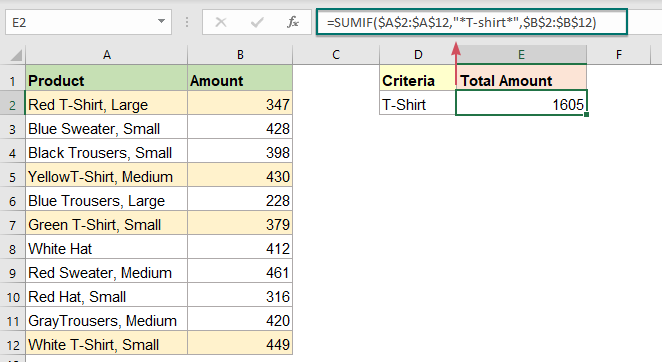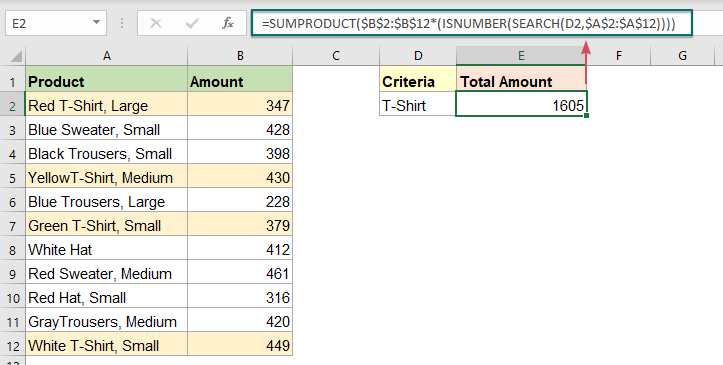## Sum if cells contain specific text in another column

This tutorial will show you how to sum values if cells contain specific or partial text in another column. Take the below data range as an example, to get the total amounts of the products containing the text “T-Shirt”, both the SUMIF function and SUMPRODUCT function can deal with this task in Excel.#### Sum values if cell contains specific or partial text with SUMIF function

To sum values if a cell contains specific text in another column, you can use the SUMIF function with a wildcard (*), the generic syntaxes are:

Generic formula with hardcoded text:

=SUMIF(range,"*text*",sum_range)
• range: The data range you want to evaluate using the criteria;
• *text*: The criteria you want to sum values based on. Here, the * wildcard is used to find any number of characters, to match all items that contain a specific text, put the text between the two * characters. (Note that you must enclose the text and wildcard in double quotes.)
• sum_range: The range of cells with matched numeric values that you want to sum.

Generic formula with cell reference:

=SUMIF(range,"*"&cell&"*",sum_range)
• range: The data range you want to evaluate using the criteria;
• "*"&cell&"*": The criteria you want to sum values based on;
• *: The wildcard which finds any number of characters.
• cell: The cell contains the specific text to look for.
• &: This concatenation operator (&) is used to join a cell reference with the asterisks.
• sum_range: The range of cells with matched numeric values that you want to sum.

After knowing the basic operation of the function, please use any one of the following formulas you need, and then press Enter key to get the result:

=SUMIF(\$A\$2:\$A\$12,"*T-shirt*",\$B\$2:\$B\$12)                     (Type the criteria manually)
=SUMIF(\$A\$2:\$A\$12,"*"&D2&"*",\$B\$2:\$B\$12)
(Use a cell reference)Note: This SUMIF function is not case-sensitive.

#### Sum values if cell contains specific or partial text with SUMPRODUCT function

The SUMPRODUCT function also can help you to finish this job without using any wildcard. Here, you should wrap the ISNUMBER and SEARCH functions inside a SUMPRODUCT function, the generic syntax is:

=SUMPRODUCT(sum_range *(ISNUMBER(SEARCH(criteria,range))))
• sum_range: The range of cells with matched numeric values that you want to sum;
• criteria: The criteria you want to sum values based on. It can be a cell reference or specific text you defined;
• range: The data range you want to evaluate using the criteria;

Please use any one of the below formulas into a blank cell, and press Enter key to return the result:

=SUMPRODUCT(\$B\$2:\$B\$12*(ISNUMBER(SEARCH("T-Shirt",\$A\$2:\$A\$12))))          (Type the criteria manually)
=SUMPRODUCT(\$B\$2:\$B\$12*(ISNUMBER(SEARCH(D2,\$A\$2:\$A\$12))))
(Use a cell reference)Explanation of this formula:

=SUMPRODUCT(\$B\$2:\$B\$12*(ISNUMBER(SEARCH("T-Shirt",\$A\$2:\$A\$12))))

• SEARCH("T-Shirt",\$A\$2:\$A\$12): The SEARCH function returns the location of the specific text “T-Shirt” from the data range A2:A12, so, you will get an array like this: {5;#VALUE!;#VALUE!;7;#VALUE!;7;#VALUE!;#VALUE!;#VALUE!;#VALUE!;7}.
• ISNUMBER(SEARCH("T-Shirt",\$A\$2:\$A\$12))= ISNUMBER({5;#VALUE!;#VALUE!;7;#VALUE!;7;#VALUE!;#VALUE!;#VALUE!;#VALUE!;7}): This ISNUMBER function is used to test for the numeric values, and returns a new array: {TRUE;FALSE;FALSE;TRUE;FALSE;TRUE;FALSE;FALSE;FALSE;FALSE;TRUE}.
• \$B\$2:\$B\$12*(ISNUMBER(SEARCH("T-Shirt",\$A\$2:\$A\$12)))= {347;428;398;430;228;379;412;461;316;420;449}*{TRUE;FALSE;FALSE;TRUE;FALSE;TRUE;FALSE;FALSE;FALSE;FALSE;TRUE}: To multiply these two arrays, the math operation forces the TRUE and FALSE values to 1s and 0s. So, the multiplication of the two arrays will be like this: {347;428;398;430;228;379;412;461;316;420;449}*{1;0;0;1;0;1;0;0;0;0;1}={347;0;0;430;0;379;0;0;0;0;449}.
• SUMPRODUCT(\$B\$2:\$B\$12*(ISNUMBER(SEARCH("T-Shirt",\$A\$2:\$A\$12)))) =SUMPRODUCT({347;0;0;430;0;379;0;0;0;0;449}):Finally, the SUMPRODUCT function sums all values in the array to get the result:1605.

#### Relative function used:

• SUMIF:
• The SUMIF function can help to sum cells based on one criterion.
• SUMPRODUCT:
• The SUMPRODUCT function can be used to multiply two or more columns or arrays together, and then get the sum of products.
• ISNUMBER:
• The Excel ISNUMBER function returns TRUE when a cell contains a number, and FALSE if not.
• SEARCH:
• The SEARCH function can help you to find the position of a specific character or substring from the given text string

#### More articles:

• Sum Smallest Or Bottom N Values
• In Excel, it is easy for us to sum a range of cells by using the SUM function. Sometimes, you may need to sum the smallest or bottom 3, 5 or n numbers in a data range as below screenshot shown. In this case, the SUMPRODUCT together with the SMALL function can help you to solve this problem in Excel.
• Sum Smallest Or Bottom N Values Based On Criteria
• In previous tutorial, we have discussed how to sum the smallest n values in a data range. In this article, we will perform a further advanced operation – to sum the lowest n values based on one or more criteria in Excel.

### The Best Office Productivity Tools

#### Kutools for Excel - Helps You To Stand Out From Crowd

 Popular Features: Find, Highlight or Identify Duplicates  |  Delete Blank Rows  |  Combine Columns or Cells without Losing Data  |  Round without Formula ... Super VLookup: Multiple Criteria  |  Multiple Value  |  Across Multi-Sheets  |  Fuzzy Lookup... Adv. Drop-down List: Easy Drop Down List  |  Dependent Drop Down List  |  Multi-select Drop Down List... Column Manager: Add a Specific Number of Columns  |  Move Columns  |  Toggle Visibility Status of Hidden Columns  |  Compare Columns to Select Same & Different Cells ... Featured Features: Grid Focus  |  Design View  |  Big Formula Bar  |  Workbook & Sheet Manager | Resource Library (Auto Text)  |  Date Picker  |  Combine Worksheets  |  Encrypt/Decrypt Cells  |  Send Emails by List  |  Super Filter  |  Special Filter (filter bold/italic/strikethrough...) ... Top 15 Toolsets:  12 Text Tools (Add Text, Remove Characters ...)  |  50+ Chart Types (Gantt Chart ...)  |  40+ Practical Formulas (Calculate age based on birthday ...)  |  19 Insertion Tools (Insert QR Code, Insert Picture from Path ...)  |  12 Conversion Tools (Numbers to Words, Currency Conversion ...)  |  7 Merge & Split Tools (Advanced Combine Rows, Split Excel Cells ...)  |  ... and more

Kutools for Excel Boasts Over 300 Features, Ensuring That What You Need is Just A Click Away...#### Office Tab - Enable Tabbed Reading and Editing in Microsoft Office (include Excel)

• One second to switch between dozens of open documents!
• Reduce hundreds of mouse clicks for you every day, say goodbye to mouse hand.
• Increases your productivity by 50% when viewing and editing multiple documents.
• Brings Efficient Tabs to Office (include Excel), Just Like Chrome, Edge and Firefox.